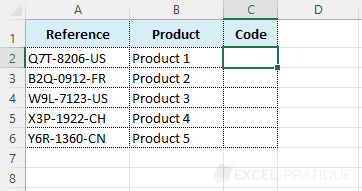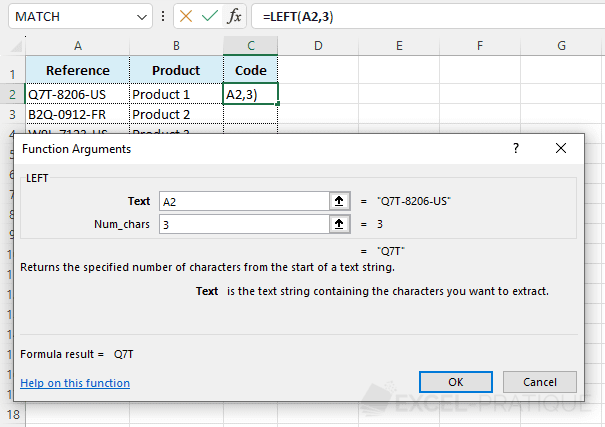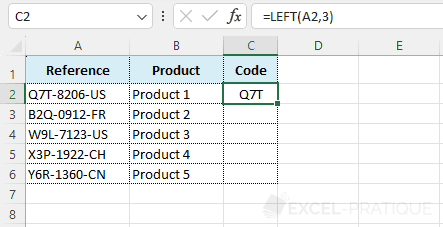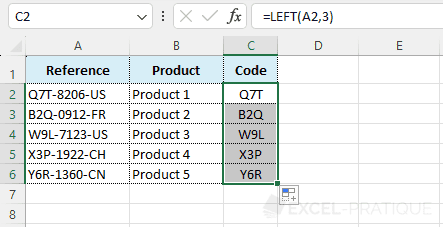# Excel Function: LEFT

The Excel function LEFT retrieves the number of characters of your choice from the left of a text string.

Usage:

`=LEFT(text, number_of_characters)`

## Example of use

The aim here is to retrieve the code that corresponds to the first 3 characters of the reference number:Select the LEFT function and enter:

• Text: the text string (here, the reference number)
• Num_chars: the number of characters to extract from the left (here, 3)
``=LEFT(A2,3)``The LEFT function indeed returns the first three characters of the reference number:Then stretch the formula down to complete the other cells: